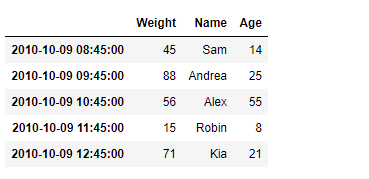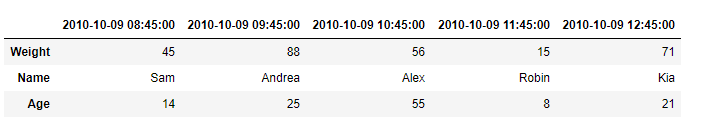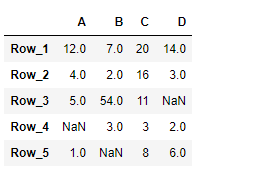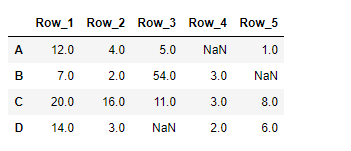# Python | Pandas DataFrame.transpose

Pandas DataFrame is a two-dimensional size-mutable, potentially heterogeneous tabular data structure with labeled axes (rows and columns). Arithmetic operations align on both row and column labels. It can be thought of as a dict-like container for Series objects. This is the primary data structure of the Pandas.

Pandas` DataFrame.transpose()` function transpose index and columns of the dataframe. It reflect the DataFrame over its main diagonal by writing rows as columns and vice-versa.

Syntax: DataFrame.transpose(*args, **kwargs)

Parameter :
copy : If True, the underlying data is copied. Otherwise (default), no copy is made if possible.
*args, **kwargs : Additional keywords have no effect but might be accepted for compatibility with numpy.

Returns : The transposed DataFrame

Example #1: Use `DataFrame.transpose()` function to find the transpose of the given dataframe.

 `# importing pandas as pd ` `import` `pandas as pd ` ` `  `# Creating the DataFrame ` `df ``=` `pd.DataFrame({``'Weight'``:[``45``, ``88``, ``56``, ``15``, ``71``], ` `                   ``'Name'``:[``'Sam'``, ``'Andrea'``, ``'Alex'``, ``'Robin'``, ``'Kia'``], ` `                   ``'Age'``:[``14``, ``25``, ``55``, ``8``, ``21``]}) ` ` `  `# Create the index ` `index_ ``=` `pd.date_range(``'2010-10-09 08:45'``, periods ``=` `5``, freq ``=``'H'``) ` ` `  `# Set the index ` `df.index ``=` `index_ ` ` `  `# Print the DataFrame ` `print``(df) `

Output :Now we will use `DataFrame.transpose()` function to find the transpose of the given dataframe.

 `# return the transpose ` `result ``=` `df.transpose() ` ` `  `# Print the result ` `print``(result) `

Output :As we can see in the output, the `DataFrame.transpose()` function has successfully returned the transpose of the given dataframe.

Example #2: Use `DataFrame.transpose()` function to find the transpose of the given dataframe.

 `# importing pandas as pd ` `import` `pandas as pd ` ` `  `# Creating the DataFrame ` `df ``=` `pd.DataFrame({``"A"``:[``12``, ``4``, ``5``, ``None``, ``1``],  ` `                   ``"B"``:[``7``, ``2``, ``54``, ``3``, ``None``],  ` `                   ``"C"``:[``20``, ``16``, ``11``, ``3``, ``8``],  ` `                   ``"D"``:[``14``, ``3``, ``None``, ``2``, ``6``]})  ` ` `  `# Create the index ` `index_ ``=` `[``'Row_1'``, ``'Row_2'``, ``'Row_3'``, ``'Row_4'``, ``'Row_5'``] ` ` `  `# Set the index ` `df.index ``=` `index_ ` ` `  `# Print the DataFrame ` `print``(df) `

Output :Now we will use `DataFrame.transpose()` function to find the transpose of the given dataframe.

 `# return the transpose ` `result ``=` `df.transpose() ` ` `  `# Print the result ` `print``(result) `

Output :As we can see in the output, the `DataFrame.transpose()` function has successfully returned the transpose of the given dataframe.

My Personal Notes arrow_drop_upCheck out this Author's contributed articles.

If you like GeeksforGeeks and would like to contribute, you can also write an article using contribute.geeksforgeeks.org or mail your article to contribute@geeksforgeeks.org. See your article appearing on the GeeksforGeeks main page and help other Geeks.

Please Improve this article if you find anything incorrect by clicking on the "Improve Article" button below.

Article Tags :

Be the First to upvote.

Please write to us at contribute@geeksforgeeks.org to report any issue with the above content.